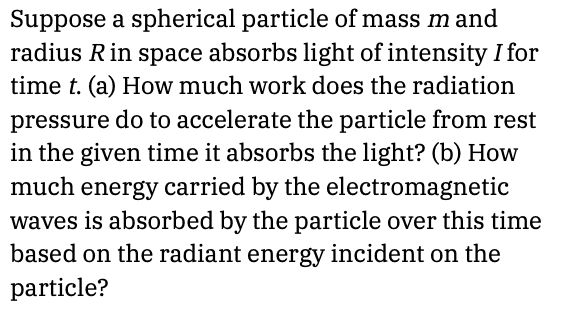# Work and energy of electromagnetic wave with intensity I

annamal
Homework Statement:
Suppose a spherical particle of mass m and radius R in space absorbs light of intensity I for time t. (a) How much work does the radiation pressure do to accelerate the particle from rest in the given time it absorbs the light? (b) How much energy carried by the electromagnetic waves is absorbed by the particle over this time based on the radiant energy incident on the particle?
Relevant Equations:
##I = \frac{P}{A}##
##P = \frac{W}{t}##
##\rho = \frac{F}{A}##
a) ##\rho = \frac{I}{c} = \frac{F}{A}## for a perfect absorber
##F = ma## where ##a = \frac{c}{t}##
##\frac{I}{c} = \frac{mc}{tA}##
##I = \frac{I^2 tA}{mc^2} = \frac{P}{A}##
##P = \frac{I^2 tA^2}{mc^2} = \frac{W}{t}##
##W = \frac{I^2 t^2A^2}{mc^2}##

I am unsure what A is. I think it should be ##4\pi r^2##, but that doesn't generate the correct answer. The correct answer also has a 0.5 factor in the work equation which I don't know how they get.

Homework Helper
Gold Member
2022 Award
I am unsure what A is. I think it should be 4πr2
If the light is being absorbed uniformly over the whole area, why would the particle move?

a=c/t? Shouldn’t that be ##a=\Delta v/t##?

Last edited:
annamal
If the light is being absorbed uniformly over the whole area, why would the particle move?
That's just how the problem is worded.
a=c/t? Shouldn’t that be ##a=\Delta v/t##?
v = c, which is the speed of light.

Homework Helper
Gold Member
2022 Award
That's just how the problem is worded.
No, it is your misinterpretation of the problem. For the problem to be solvable you will have to assume the light is coming from a distant point source.
v = c, which is the speed of light.
You wrote F=ma. m is given as the mass of the particle. It does not accelerate to the speed of light.

annamal
No, it is your misinterpretation of the problem. For the problem to be solvable you will have to assume the light is coming from a distant point source.
See below. It is worded exactly asYou wrote F=ma. m is given as the mass of the particle. It does not accelerate to the speed of light.
What does it accelerate to then?

Homework Helper
Gold Member
2022 Award
See below. It is worded exactly as
Nowhere does it specify where the radiation is coming from. If it were uniformly from all directions there would be no net force on the particle so it would not move.
So you will have to assume it is all coming from the same direction. Can you work out from that what area to use?
What does it accelerate to then?
Your first task is to find the force exerted. From that you can get the acceleration, and the speed that it reaches and the distance that it travels in time t. Then you can calculate the work done on the particle and the KE achieved.

What I am unsure about is whether you are supposed to consider the decline in radiation pressure as the particle gathers speed. Is this part of a relativity course?

Last edited:
annamal
Nowhere does it specify where the radiation is coming from. If it were uniformly from all directions there would be no net force on the particle so it would not move.
So you will have to assume it is all coming from the same direction. Can you work out from that what area to use?

Your first task is to find the force exerted. From that you can get the acceleration and speed it reaches and distance it travels in time. Then you can calculate the work done on the particle and the KE achieved.

What I am unsure about is whether you are supposed to consider the decline in radiation pressure as the particle gathers speed. Is this part of a relativity course?
No it's not.